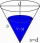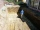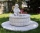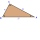# Ratio of sides

Calculate the area of a circle that has the same circumference as the circumference of the rectangle inscribed with a circle with a radius of r 9 cm so that its sides are in ratio 2 to 7.

Correct result:

S =  157.617 cm2

#### Solution:

$r=9 \ \text{cm} \ \\ r^2=(a/2)^2 + (b/2)^2 \ \\ a:b=2:7 \ \\ \ \\ r^2=(a/2)^2 + ((7/2 \cdot \ a)/2)^2 \ \\ r^2=a^2/4 + a^2 \cdot \ (7/4)^2 \ \\ \ \\ a=r / (\sqrt{ 1/4+(7/4)^2 })=9 / (\sqrt{ 1/4+(7/4)^2 }) \doteq 4.945 \ \text{cm} \ \\ \ \\ b=7/2 \cdot \ a=7/2 \cdot \ 4.945 \doteq 17.3074 \ \text{cm} \ \\ \ \\ o=2 \cdot \ (a+b)=2 \cdot \ (4.945+17.3074) \doteq 44.5048 \ \text{cm} \ \\ \ \\ o=2 \pi \cdot \ r_{1} \ \\ \ \\ r_{1}=o / (2 \pi)=44.5048 / (2 \cdot \ 3.1416) \doteq 7.0832 \ \text{cm} \ \\ \ \\ \ \\ S=\pi \cdot \ r_{1}^2=3.1416 \cdot \ 7.0832^2=157.617 \ \text{cm}^2$Our examples were largely sent or created by pupils and students themselves. Therefore, we would be pleased if you could send us any errors you found, spelling mistakes, or rephasing the example. Thank you!

Please write to us with your comment on the math problem or ask something. Thank you for helping each other - students, teachers, parents, and problem authors.Tips to related online calculators
Looking for help with calculating roots of a quadratic equation?
Check out our ratio calculator.
Pythagorean theorem is the base for the right triangle calculator.

#### You need to know the following knowledge to solve this word math problem:

We encourage you to watch this tutorial video on this math problem:

## Next similar math problems:

• Ratio of squaresA circle is given in which a square is inscribed. The smaller square is inscribed in a circular arc formed by the side of the square and the arc of the circle. What is the ratio of the areas of the large and small squares?
• Square and circlesThe square in the picture has a side length of a = 20 cm. Circular arcs have centers at the vertices of the square. Calculate the areas of the colored unit. Express area using side a.
• Hexagonal pyramidCalculate the volume and surface area of a regular hexagonal pyramid with a base edge a = 30 m and a side edge b = 50 m.In a regular quadrilateral pyramid, the height is 6.5 cm and the angle between the base and the side wall is 42°. Calculate the surface area and volume of the body. Round calculations to 1 decimal place.
• Four prismsQuestion No. 1: The prism has the dimensions a = 2.5 cm, b = 100 mm, c = 12 cm. What is its volume? a) 3000 cm2 b) 300 cm2 c) 3000 cm3 d) 300 cm3 Question No.2: The base of the prism is a rhombus with a side length of 30 cm and a height of 27 cm. The heigWhat is the volume of a quadrilateral oblique prism with base edges of length a = 1m, b = 1.1m, c = 1.2m, d = 0.7m, if a side edge of length h = 3.9m has a deviation from the base of 20° 35 ´ and the edges a, b form an angle of 50.5°.
• Hexagonal pyramidFind the volume of a regular hexagonal pyramid, the base edge of which is 12 cm long and the side edge 20 cm.A regular quadrilateral pyramid has a volume of 24 dm3 and a base edge a = 4 dm. Calculate: a/height of the pyramid b/sidewall height c/surface of the pyramid
• Edge of prismThe regular quadrilateral prism has a surface of 250 dm2, its shell has a content of 200 dm2. Calculate its leading edge.
• The regularThe regular triangular prism has a base in the shape of an isosceles triangle with a base of 86 mm and 6.4 cm arms, the height of the prism is 24 cm. Calculate its volume.
• The funnelThe funnel has the shape of an equilateral cone. Calculate the content of the area wetted with water if you pour 3 liters of water into the funnel.
• The trenchCalculate how many cubic meters of soil needs to be removed from the excavation in the shape of an isosceles trapezoid, the top width is 3 meters, the lower width is 1.8 m, the depth of the excavation is 1 m, and the length is 20 m.
• FountainThe stone fountain, which has the shape of a cylinder with a diameter of 3 m, is 70 cm deep. How many m2 of stone is wetted with water?
• Hexagonal pyramidFind the area of a shell of the regular hexagonal pyramid, if you know that its base edge is 5 cm long and the height of this pyramid is 10 cm.
• HeightThe content of the triangle is 35 cm2. The length of the base is 10 cm. Determine the length of the height on the base.
• Dimensions of the trapezoidOne of the bases of the trapezoid is one-fifth larger than its height, the second base is 1 cm larger than its height. Find the dimensions of the trapezoid if its area is 115 cm2
• Cylinder containerThe cylindrical container with a diameter of 1.8 m contains 2,000 liters of water. How high does the water reach?